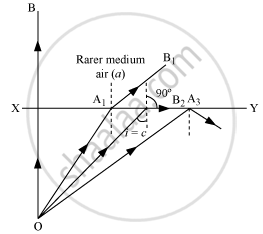# Answer the Following Question. Under What Conditions is the Phenomenon of Total Internal Reflection of Light Observed? Obtain the Relation Between the Critical Angle of Incidence. - Physics

Under what conditions is the phenomenon of total internal reflection of light observed? Obtain the relation between the critical angle of incidence and the refractive index of the medium.

#### Solution

Total Internal Reflection:Total internal reflection is the phenomenon of reflection of light into a denser medium from an interface of the denser medium and the rarer medium. Two essential conditions for total internal reflection: Incident ray should travel in the denser medium and refracted ray should travel in the rarer medium.
The angle of incidence (i) should be greater than the critical angle for the pair of media in contact.
The relation between refractive index and critical angle (C):
When i = C and r = 90°
Apply Snell's law

mu_b sinC = mu_a sin90^circ = mu_a xx 1

mu_b/mu_a = 1/sin C

""^amu_b = 1/sin C.

Concept: Reflection of Light by Spherical Mirrors
Is there an error in this question or solution?